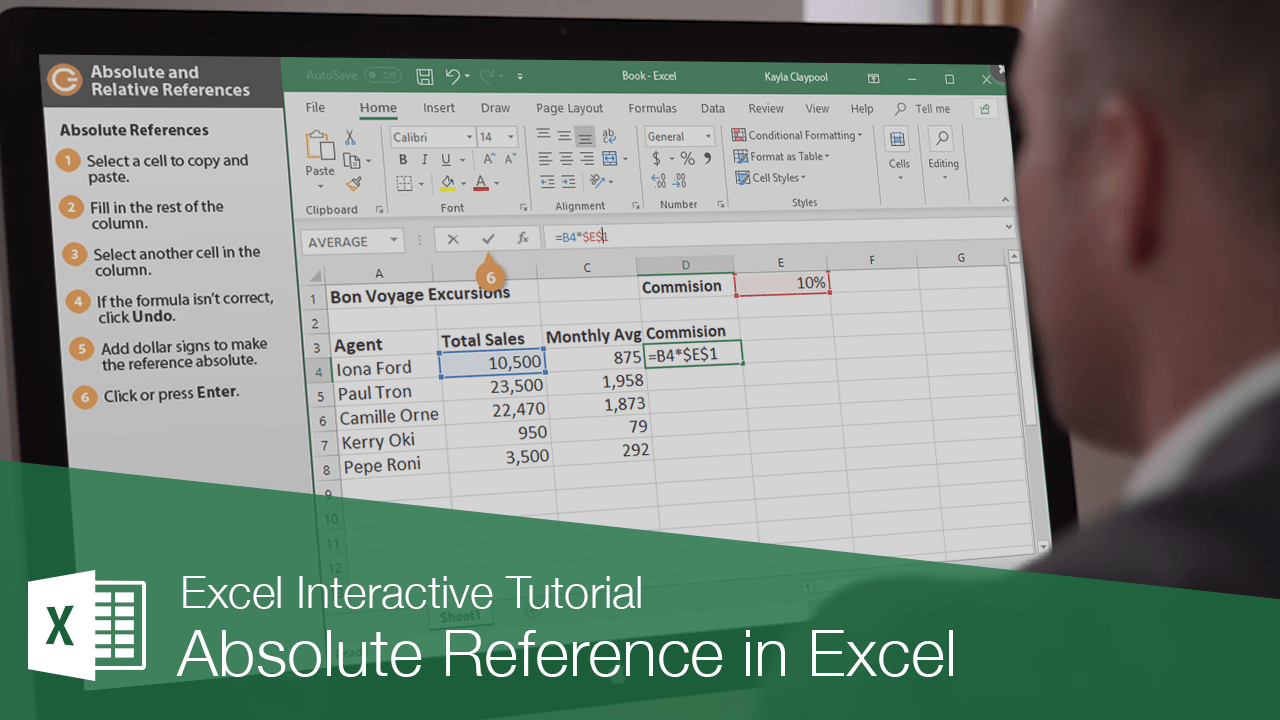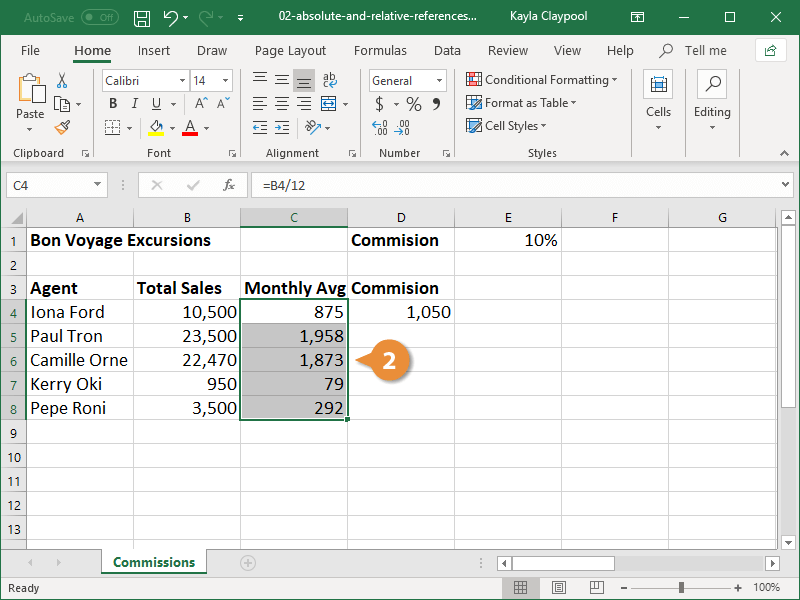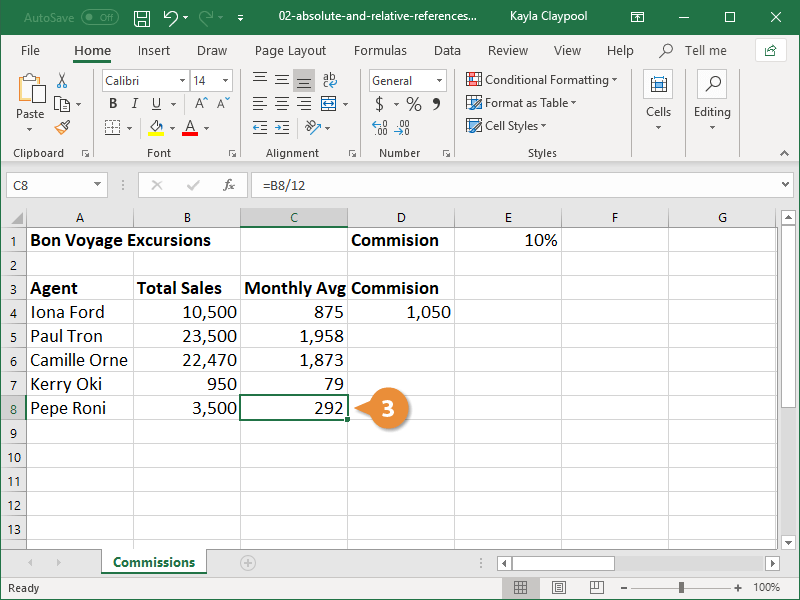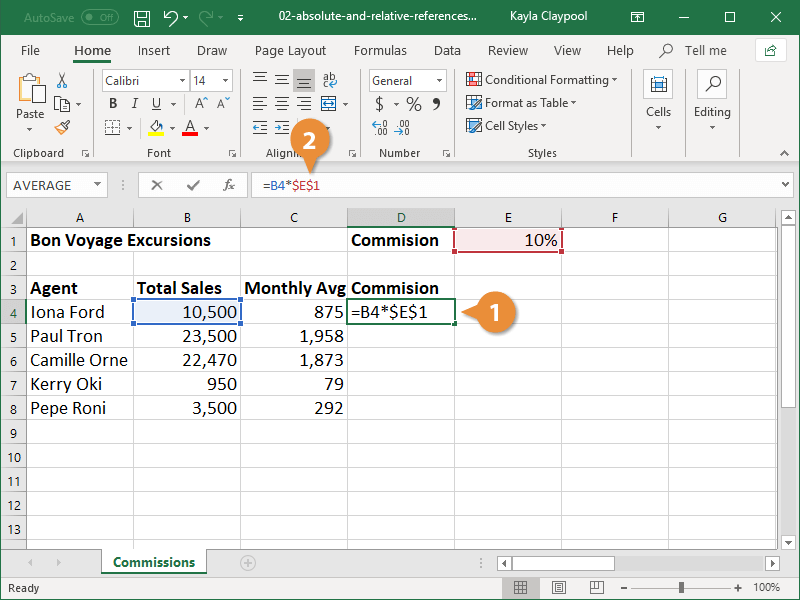Absolute Reference in Excel | CustomGuide# Absolute Reference in Excel

How to use Absolute and Relative Cell References in ExcelFormulas can contain numbers, like 5 or 8, but more often they reference the contents of cells. A cell reference tells Excel where to look for values you want to use in a formula. For example, the formula =A5+A6 adds the values in cells A5 and A6.

Using cell references is useful because if you change the values in the referenced cells, the formula result automatically updates using the new values. There are two types of cell references: relative and absolute.

### Relative References

Relative references refer to cells in relation to the location of the cell that contains the formula. When the formula is moved, it references new cells based on their location. Relative references are the default type of references in Excel.

1. Copy the formula in an existing cell that you want to paste elsewhere in the worksheet.
2. Paste the formula in the desired cells.3. Select a cell that contains the pasted formula. Use the formula bar to verify the cell references have updated relative to where the formula was pasted.In the cell(s) where the formula was pasted, the cell reference updates for the current row and/or column.

### Absolute References

Absolute references always refer to the same cell, even when the formula is copied and pasted. Absolute references are indicated with dollar signs in formulas (e.g. \$A\$1).

1. Select the cell that contains a cell reference you want to convert to an absolute reference.
2. In the formula bar, add dollar signs (\$) to the reference you want to remain absolute.• Absolute column and row reference (\$A\$1): The column and row remain constant no matter where the formula is pasted.
• Absolute column reference (\$A1): The column remains absolute no matter where the formula is pasted, but the row updates relatively.
• Absolute row reference (A\$1): The row remains absolute no matter where the formula is pasted, but the column updates relatively.

Click in any cell reference in the formula bar and press F4 to convert it to an absolute reference. Continue to press F4 to change the type of absolute reference.